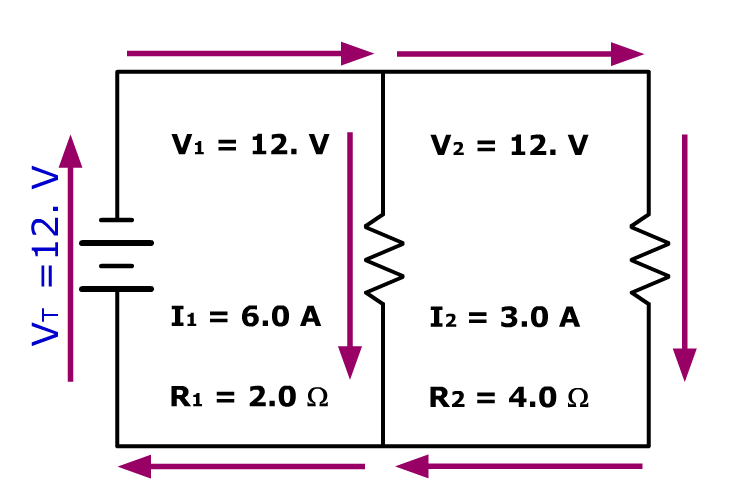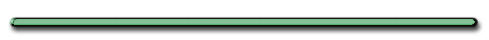A pool filled with non-newtonian fluid

 You can make this fluid with 2 ingredients found  in any kitchen!!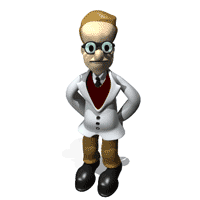It is nothing more than water & cornstarch! It solidifies with pressure.Parallel Circuit Practice ProblemsTotal Resistance Parallel Circuit

Circuits & Total Resistance - Flash

showmethephysics.com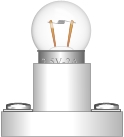Learning-Connections Clipart

Deriving an Equation for Total Resistance

Since IT = I1 + I2 + I3 + I4 +……

Then …

 VT = V1 + V2 + V3 RT R1 R2 R3

On Reference Table

 1 = 1 + 1 + 1 RT R1 R2 R3RT - Total Resistance

A.K.A.

"Equivalent Resistance"
"Combined Resistance"
"Effective Resistance"Ex 3) Find the total resistance for 3 resistors of 2.0 ohms, 5.0 ohms and 2.0 ohms connected in parallel.

 1 = 1 + 1 + 1 RT R1 R2 R3

 1 = 1 + 1 + 1 RT 2.0 Ω 5.0 Ω 2.0 Ω

Find common denominator and solve

1/RT = 5/10 Ω + 2/10 Ω + 5/10 Ω

1/RT = (5 + 2 + 5)/10

1/RT = 12/10

RT = 10/12 ohms

RT = .83 ohms** The more resistors
connected in parallel
the smaller the total resistance!!!

[ HTML5 ]
St. Mary's H.S.

Why?

Every time you add a resistor in parallel,

you add another branch for current,

so you create more current.

This reduces the total RTotal Resistance Equation #2
for parallel circuit

 RT = VT/ITEx 4) Resistors of 3.0 Ω, 6.0 Ω, 9.0 Ω are connected in parallel.

Find combined R

 1 = 1 + 1 + 1 RT R1 R2 R3

1/RT =

1/3.0 Ω + 1/6.0 Ω + 1/9.0 Ω

(common denominator, 18)

= (6.0 + 3.0 + 2.0)/18. = 11./18.

RT = 18./11. Ω

= 1.6 ohmsEx. 5)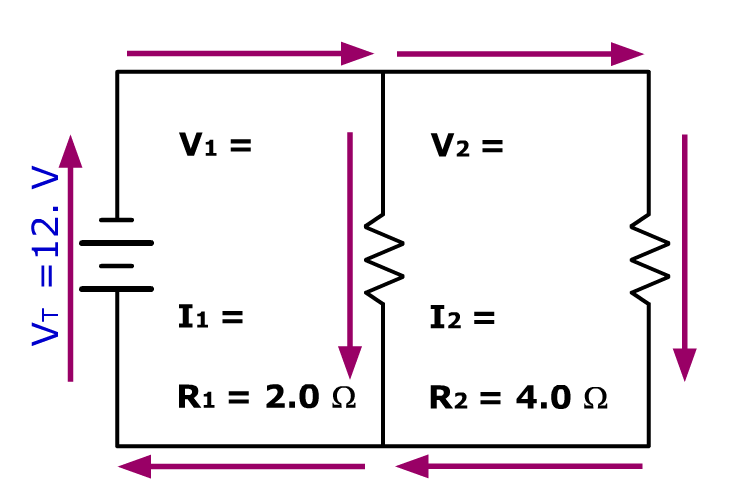a) Find the equivalent resistance for this parallel circuit

 1 = 1 + 1 + 1 RT R1 R2 R3

1/RT  = 1/2.0 Ω + 1/4.0 Ω

1/RT = 3.0 Ω/4.0 Ω

RT =4.0 Ω/3.0 Ω

= 1.3 ohms

b) Find IT (Current coming out of the source)VT  = ITRT

12. V = IT(1.3 ohms)

IT = 9.0 ampsc) Find the potential drop (V) across R1 & R2

 VT = V1 = V2 = V3

VT = V1 = V2 = 12V